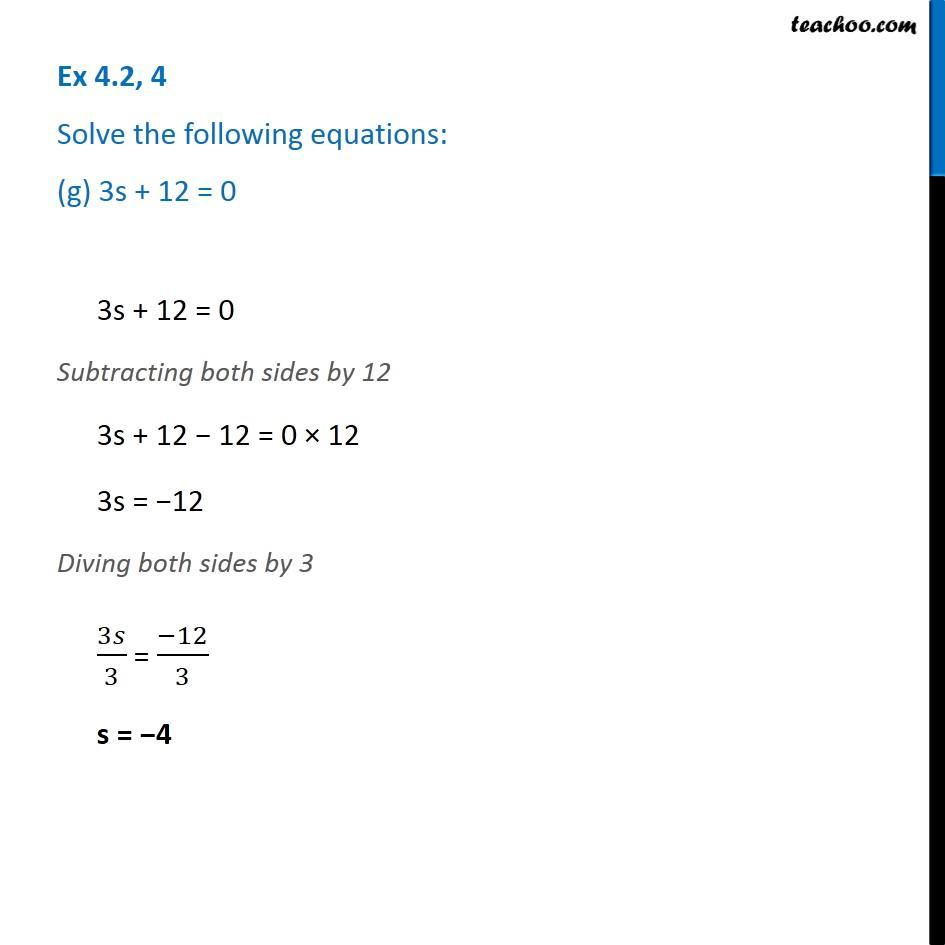Ex 4.2

Chapter 4 Class 7 Simple Equations
Serial order wiseLearn in your speed, with individual attention - Teachoo Maths 1-on-1 Class

### Transcript

Ex 4.2, 4 Solve the following equations: (g) 3s + 12 = 0 3s + 12 = 0 Subtracting both sides by 12 3s + 12 − 12 = 0 × 12 3s = −12 Diving both sides by 3 3𝑠/3 = (−12)/3 s = −4